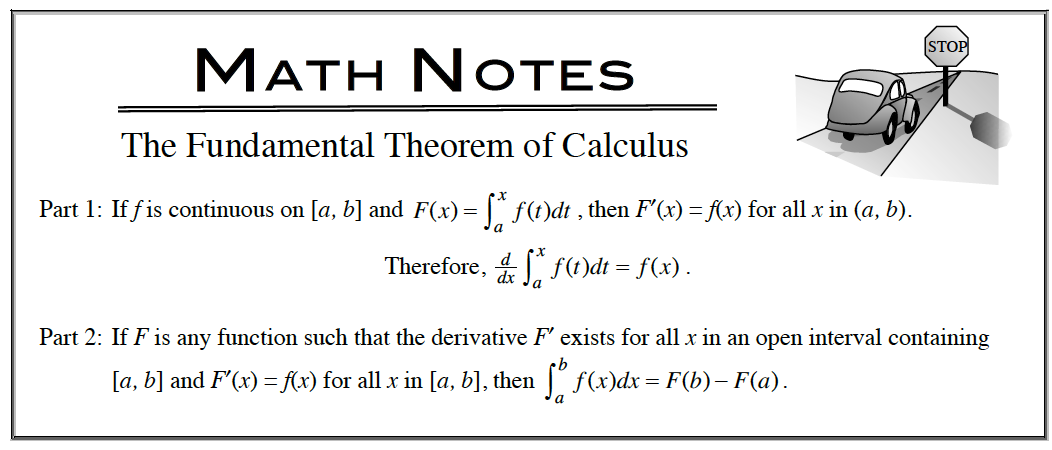Home > APCALC > Chapter 7 > Lesson 7.4.1 > Problem7-184

7-184.

Evaluate. Homework Help ✎

All of these problems involve the Fundamental Theorem of Calculus, part 1.

1. $\frac { d } { d x } \int ( 3 x ^ { 2 } + \operatorname { sin } ( x ^ { 2 } ) ) d x$2. $\int \frac { d } { d x } ( 3 x ^ { 2 } + \operatorname { sin } ( x ^ { 2 } ) ) d x$

Compare and contrast part (a) with part (b). The answers will NOT be identical.

3. $\frac { d } { d x } \int _ { - 1 } ^ { \operatorname { cos } ( x ) } ( 3 x ^ { 2 } + \operatorname { sin } ( x ^ { 2 } ) ) d x$

The Fundamental Theorem of Calculus, part 1 states that the derivative of an INDEFINITE integral is the same as the original function.
But this is a DEFINITE integral: Remember to multiply by the derivative of the bounds.

4. $\frac { d } { d x } \int _ { \operatorname { ln } ( x ) } ^ { \operatorname { cos } ( x ) } ( 3 x ^ { 2 } + \operatorname { sin } ( x ^ { 2 } ) ) d x$